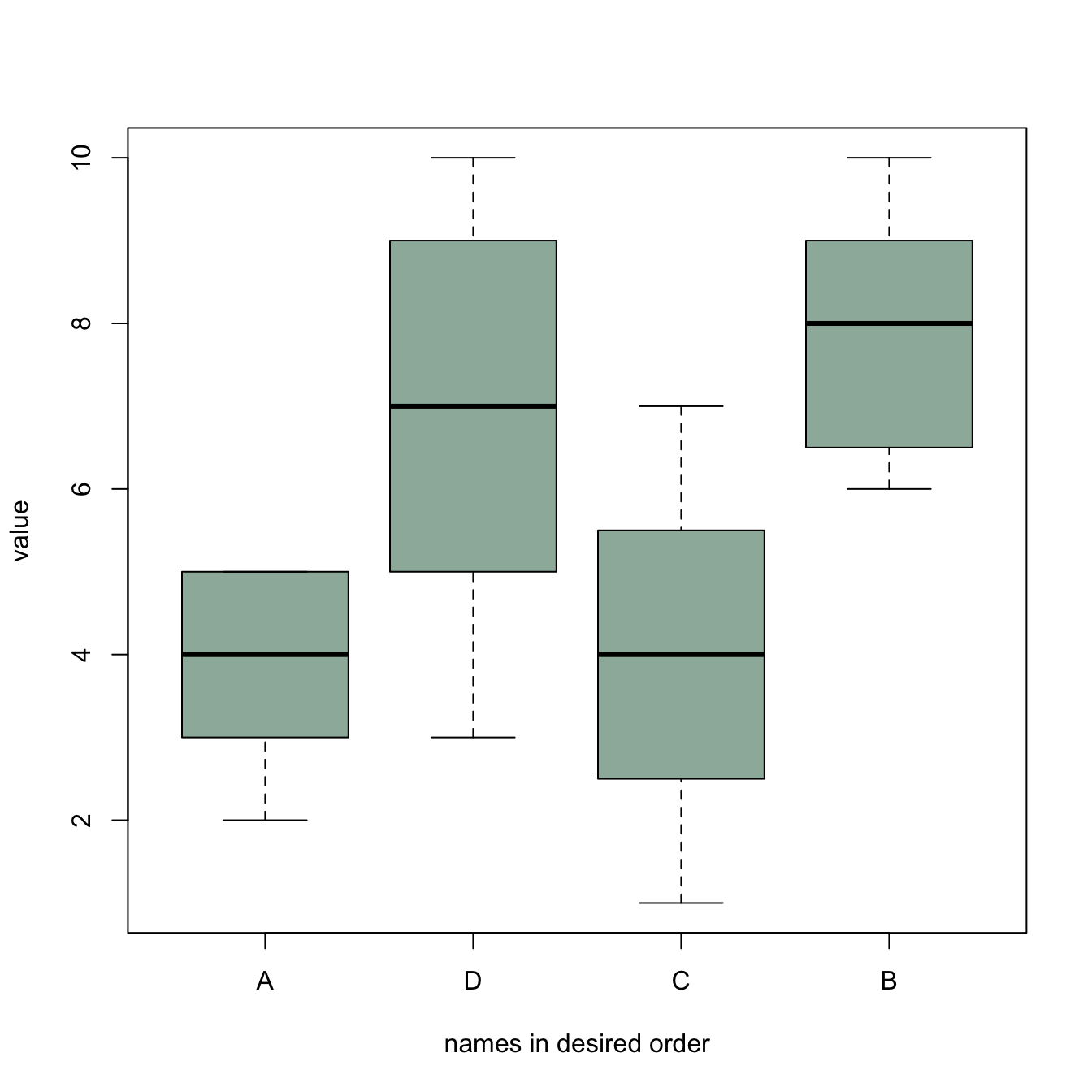# Specific order for boxplot categories

This example explains how to build a boxplot with base R, sorting groups in a specific order.

Boxplot categories are provided in a column of the input data frame. This column needs to be a `factor`, and has several `levels`. Categories are displayed on the chart following the order of this factor, often in alphabetical order.

Sometimes, we need to show groups in a specific order (A,D,C,B here). This can be done by reordering the levels, using the `factor()` function.

Note: A complete post about reordering factors is available here.``````#Creating data
names <- c(rep("A", 20) , rep("B", 20) , rep("C", 20), rep("D", 20))
value <- c( sample(2:5, 20 , replace=T) , sample(6:10, 20 , replace=T),
sample(1:7, 20 , replace=T), sample(3:10, 20 , replace=T) )
data <- data.frame(names,value)

# Classic boxplot (A-B-C-D order)
# boxplot(data\$value ~ data\$names)

# I reorder the groups order : I change the order of the factor data\$names
data\$names <- factor(data\$names , levels=c("A", "D", "C", "B"))

#The plot is now ordered !
boxplot(data\$value ~ data\$names , col=rgb(0.3,0.5,0.4,0.6) , ylab="value" ,
xlab="names in desired order")``````

Related chart types

## Contact

This document is a work by Yan Holtz. Any feedback is highly encouraged. You can fill an issue on Github, drop me a message on Twitter, or send an email pasting yan.holtz.data with gmail.com.